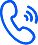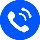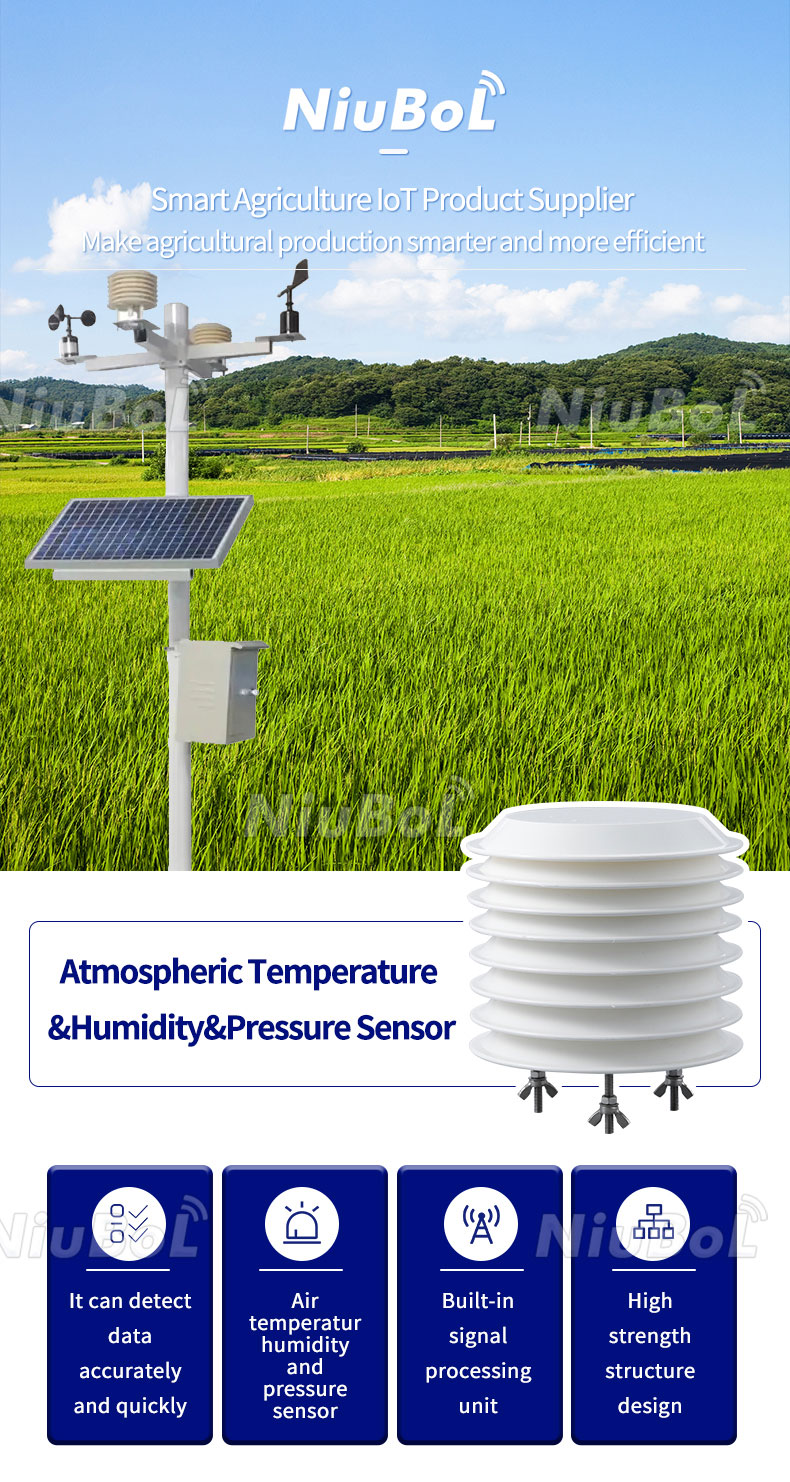Hotline：+8618073152920— Blogs —

—Products—Consumer hotline +8618073152920
Changsha Zoko Link Technology Co., Ltd.

Email：Arvin@niubol.com

WhatsApp：+8615367865107

Address：Room 102, District D, Houhu Industrial Park, Yuelu District, Changsha City, Hunan Province, China

Position：Home >> Blogs >> Technical Support

Technical Support

# Temperature and humidity sensor output 4-20mA analogue conversion formula

Time：2023-10-18 15:34:16 Popularity：130

Current signal output 4-20mA signal, if the actual measurement is 12 mA, the sensor temperature range is -40 ℃ ~ 80 ℃, then calculate the temperature is how many degrees?

A: First of all, you need to convert the 4-20mA current signal to a temperature signal, which requires knowing the relationship between temperature and current. This relationship depends on the type of temperature sensor used. Common types of temperature sensors include thermocouples, thermistors and semiconductor temperature sensors. Assuming that a thermistor temperature sensor is used, the following equation can be used to convert the current signal to a temperature signal:

Temperature = (Current value - 4mA) / (16mA) * (Upper range limit - Lower range limit) + Lower range limit

Where the current value is the actual measured current value, the lower range limit is -40°C, and the upper range limit is 80°C. Substitute the actual measured current value of 12mA into the formula.

Temperature conversion formula: (12mA - 4mA) / (16mA) * (80°C - (-40°C)) +(- 40°C)

First calculate the numerator: 8

Then calculate the denominator: 16

Then calculate the temperature range: 120℃

Finally bring in the formula to calculate the result:

(8/16) × 120-40 = 20°C

Therefore, the measured 12mA current signal corresponds to a temperature of 20℃.Current signal output 4-20mA signal, if the actual measurement of 12mA, the sensor humidity range is 0-100RH%, then counted into the humidity is how much?

Humidity = (current value - 4mA) / (16mA) * (upper range limit - lower range limit) + lower range limit

Humidity conversion formula: (12mA - 4mA) / (16mA) * (100RH - 0RH)) +(0RH)

First calculate the numerator: 8

Then calculate the denominator: 16

Then calculate the Humidity range: 100RH

Finally bring in the formula to calculate the result:

Humidity value = (12-4)/16*100+0 =50RH%

Related recommendations

Related products

Name*

Tel*

Email*

Company

Country

Message

online
Contacts
Email
Top
XScreenshot, WhatsApp to identify the QR code

WhatsApp number：+8615367865107

（Click on WhatsApp to copy and add friends）

Open WhatsApp

The WhatsApp ID has been copied, please open WhatsApp to add consultation details!